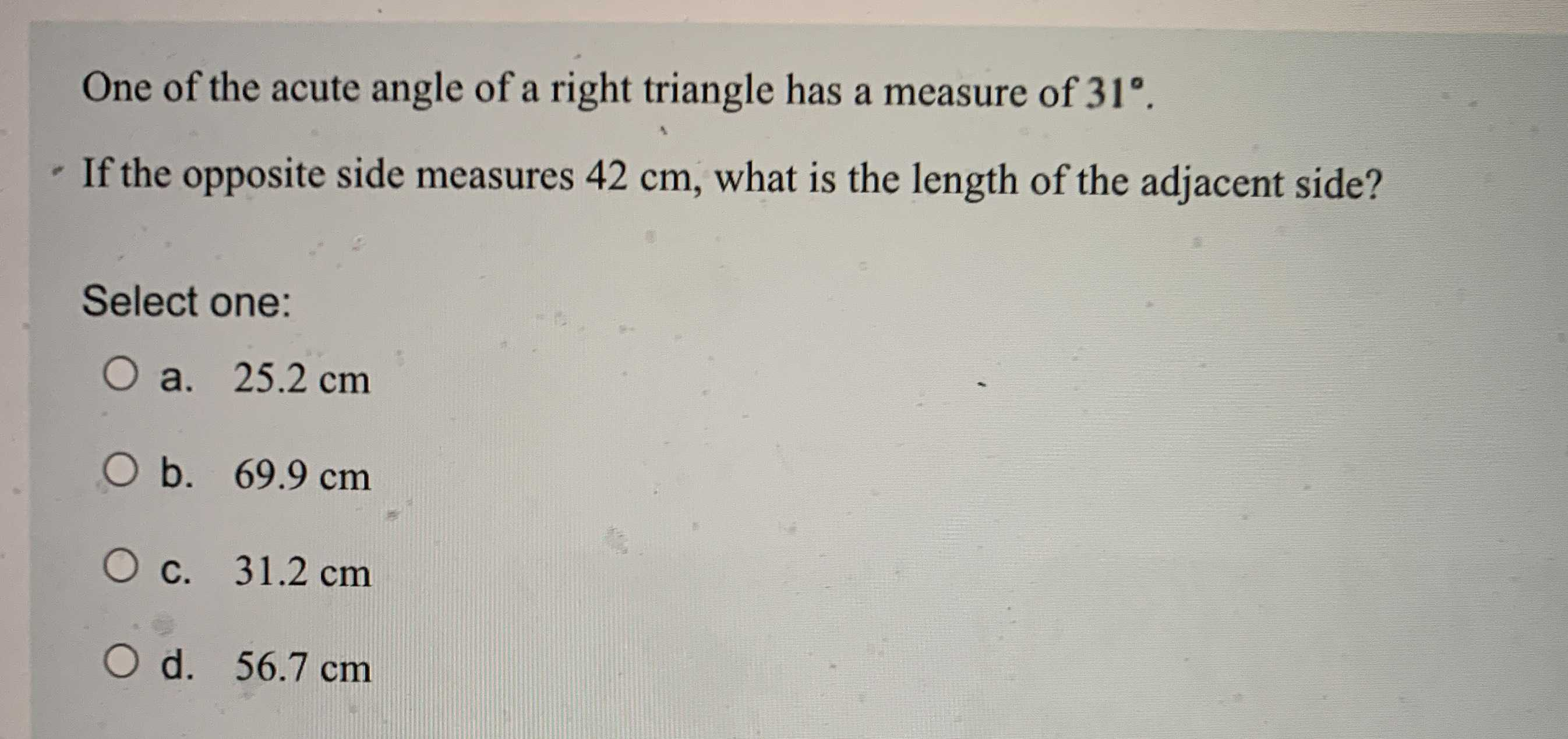### Still have math questions?

Trigonometry
QuestionOne of the acute angle of a right triangle has a measure of $$31 ^ { \circ }$$ .

If the opposite side measures $$42 cm$$ , what is the length of the adjacent side? Select one:

a. $$25.2 cm$$

b. $$69.9 cm$$

c. $$31.2 cm$$

d. $$56.7 cm$$

adjacent side = $$\frac{42}{tan31}= 69.9 cm$$